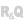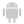HelpWLanguageWLanguage functionsControls, pages and windowsDiagramShapeToImagePresentationExampleSee alsoDiagram constantsDiagram functionsDiagramEndModificationDiagramGroupDiagramInfoXYDiagramLoadDiagramLoadLibraryDiagramSaveDiagramSearchDiagramSelectMinusDiagramSelectPlusDiagramShapeToImageDiagramStartModificationDiagramToImageDiagramToSVGDiagramUngroup
 WINDEVWEBDEVWINDEV MobileOthersDiagramShapeToImage (Function) In french: DiagrammeFormeVersImage Creates an image from a shape in a diagram (the shape can be displayed or not in the diagram). This function can be used to create a thumbnail of a shape, for example. Example // Display the preview of a shapeMyShape is diagRectangleMyShape.Width = 320MyShape.Height = 200MyImage is Image MyImage = DiagramShapeToImage(MyShape, 64, 64, 8)IMG_Preview = MyImage Syntax = DiagramShapeToImage( , , [, ]) : Image variableImage variable that corresponds to the desired shape. : diagShape variableName of the diagShape variable (or one of its derivatives) to be used. You can use the following variables: diagPath variable, diagGroup variable, diagImage variable, diagConnector variable, diagOval variable, diagPolygon variable, diagRectangle variable. : RealTotal width (in pixels) of the image to be created. : RealTotal height (in pixels) of the image to be created. : Optional realSize of the frame around the shape (in pixels)Component: wd280mdl.dll Minimum version required Version 27 This page is also available for…